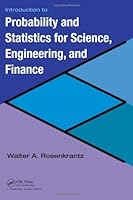# Introduction to Probability and Statistics for Science, Engineering, and Finance## Book Description

Integrating interesting and widely used concepts of financial engineering into traditional courses, Introduction to Probability and Statistics for Science, Engineering, and Financeillustrates the role and scope of statistics and probability in various fields.

The text first introduces the basics needed to understand and create tables and produced by standard statistical packages, such as Minitab, SAS, and JMP. It then takes students through the traditional topics of a first course in statistics. Novel features include:

• Applications of standard statistical concepts and methods to the analysis and interpretation of financial data, such as risks and returns
• Cox–Ross–Rubinstein (CRR) model, also called the binomial lattice model, of stock price fluctuations
• An application of the central limit theorem to the CRR model that yields the lognormal distribution for stock prices and the famous Black–Scholes option pricing formula
• An introduction to modern theory
• Mean-standard deviation diagram of a collection of portfolios
• a stock’s betavia simple linear regression

As soon as he develops the statistical concepts, the author presents applications to engineering, such as queuing theory, reliability theory, and acceptance sampling; ; public health; and finance. Using both statistical software packages and scientific calculators, he reinforces fundamental concepts with numerous examples.

## Book Details

• Title: Introduction to Probability and Statistics for Science, Engineering, and Finance
• Author:
• Length: 680 pages
• Edition: 1
• Language: English
• Publisher:
• Publication Date: 2008-07-10
• ISBN-10: 1584888121
• ISBN-13: 9781584888123